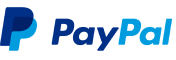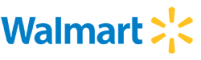New update is available. Click here to update.
Topics

# Best Time to Buy and Sell

Moderate0/80
Average time to solve is 20m+12 more companies

## Problem statement

You are given an array(PRICES) of stock prices for N consecutive days. Your task is to find the maximum profit that you can make by completing as many transactions as you like, where a transaction denotes buying one and selling one share of the stock.

Note:

``````You must sell the stock before you buy it again.
``````
Detailed explanation ( Input/output format, Notes, Images )
Constraints:
``````1 <= N <= 5 * 10^4
0 <= PRICES[i] <= 10^4

Time Limit: 1sec
``````
Sample Input 1:
``````6
2 4 7 1 3 5
``````
Sample Output 1:
``````9
``````
Explanation for Sample Input 1:
``````As we are allowed to do any number of transactions to maximize the profit,
The first transaction we will do is to buy on day 1 (PRICE = 2) and sell on day 3 (PRICE = 7), making a profit of  5 (7 - 2).
The second transaction we will do is to buy on day 3 (PRICE = 1) and sell on day = 6 (PRICE = 5), making a profit of 4 (5 - 1).
Total profit = 5 + 4 = 9.
``````
Sample Input 2:
``````4
1 2 3 4
``````
Sample Output 2:
``````3
``````Console